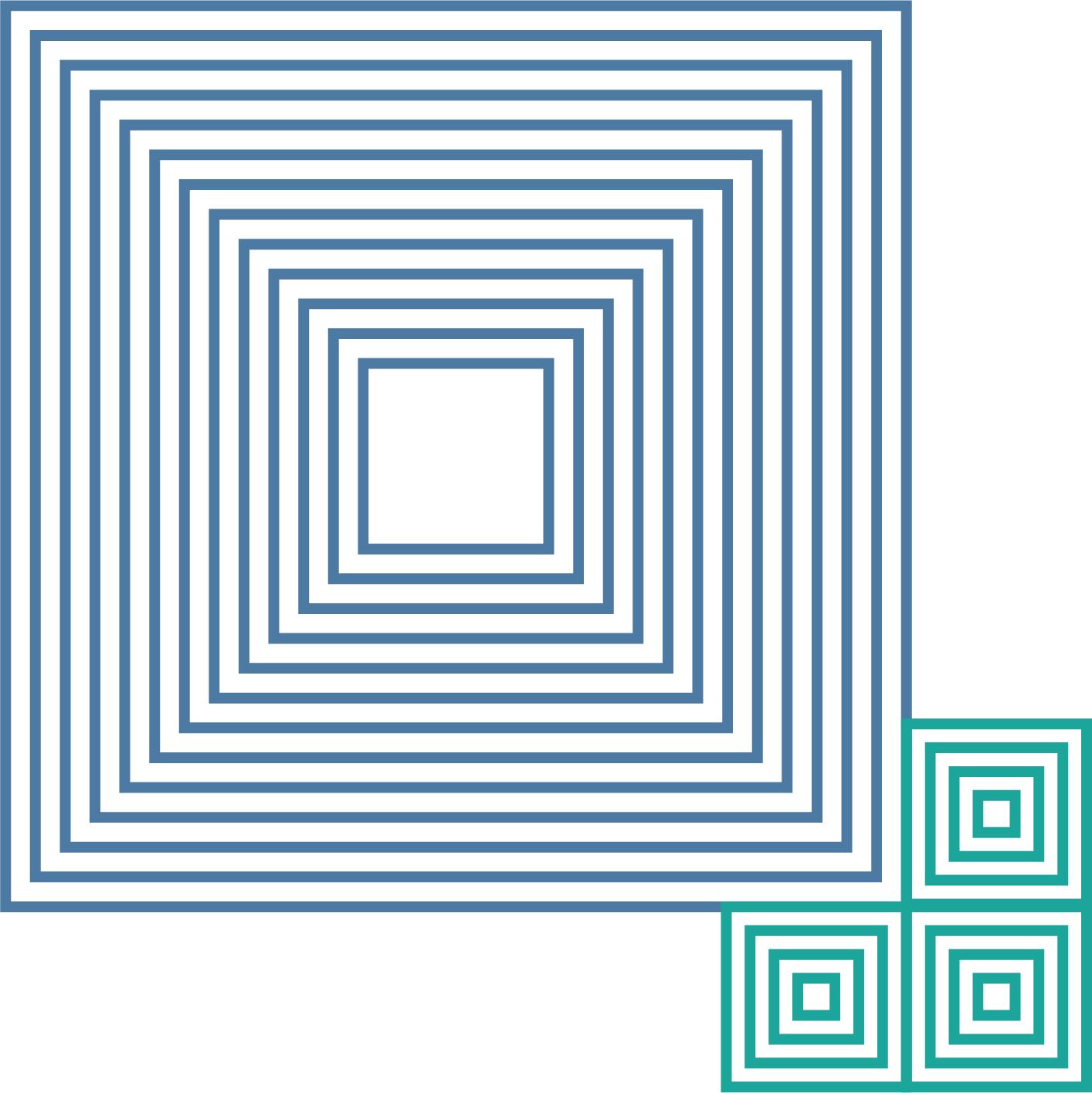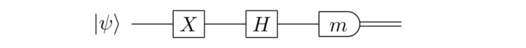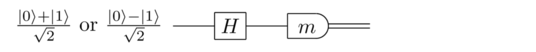QUANTUM COMPUTING THE SOFT WAY

# Quantum Measurements

What are "measurements" in quantum physics, and how are they different from physical measurement in classical physics? This chapter is an exploration into this question. We begin by listening to the words of Prof. Paola Verrucchi, from the University of Florence, who tells us how she would explain quantum measurements to her grandma.

Now, let's focus on measuring our quantum bits. Suppose a (hypothetical!) quantum physicist named Alice prepares a qubit in her laboratory, in a quantum state $\alpha|0\rangle+\beta|1\rangle$. Then she gives her qubit to another quantum physicist, Bob, but doesn’t tell him the values of $\alpha$ and $\beta$. Is there some way Bob can figure out $\alpha$ and $\beta$? That is, is there some experiment Bob can do to figure out the identity of the quantum state?

The surprising answer to this question turns out to be NO! There is, in fact, no way to figure out $\alpha$ and $\beta$ if they start out unknown. To put it a slightly different way, the quantum state of any system – whether it be a qubit or a some other system – is not directly observable.

I say this is surprising, because it’s very different from our usual everyday way of thinking about how the world works. If there’s something wrong with your car, a mechanic can use diagnostic tools to learn about the internal state of the engine. The better the diagnostic tools, the more they can learn. Of course, there may be parts of the engine that would be impractical to access – maybe they’d have to break a part, or use a microscope, for instance. But you’d probably be rather suspicious if the mechanic told you the laws of physics prohibited them from figuring out the internal state of the engine.

Similarly, when you first start learning about quantum circuits, it seems like we should be able to observe the amplitudes of a quantum state whenever we like. But that turns out to be prohibited by the laws of physics. Those amplitudes are better thought of as a kind of hidden information.

So, what can we figure out from the quantum state? Rather than somehow measuring $\alpha$ and $\beta$, there are other ways of getting useful information out of a qubit. Let me describe an especially important process called measurement in the computational basis. This is a fundamental primitive in quantum computing: it’s the way we typically extract information from our quantum computers. I’ll explain now how it works for a single qubit, and later generalize to multi-qubit systems.

Suppose a qubit is in the state $\alpha|0\rangle+\beta|1\rangleα$. When you measure this qubit in the computational basis it gives you a classical bit of information: it gives you the outcome 0 with probability $|\alpha|^2$, and the outcome 1 with probability $|\beta|^2$.

To think a little more concretely about this process, suppose your qubit is instantiated in some physical system. Perhaps it’s being stored in the state of an atom somehow. It doesn’t matter exactly what, but you have this qubit in your laboratory. And you have some measurement apparatus, probably something large and complicated, maybe involving lasers and microprocessors and a screen for readout of the measurement result. And this measurement apparatus interacts in some way with your qubit.

After the measurement interaction, your measurement apparatus registers an outcome. For instance, it might be that you get the outcome 0. Or maybe instead you get the outcome 1. The crucial fact is that the outcome is ordinary classical information – the stuff you already know how to think about – which you can then use to do other things, and to control other processes.

So the way a quantum computation works is that we manipulate a quantum state using a series of quantum gates, and then at the end of the computation (typically) we do a measurement to read out the result of the computation. If our quantum computer is just a single qubit, then that result will be a single classical bit. If, as is more usually the case, it’s multiple qubits, then the measurement result will be multiple classical bits.

A fundamental fact about this measurement process is that it disturbs the state of the quantum system. In particular, it doesn’t just leave the quantum state alone. After the measurement, if you get the outcome 0 then the state of the qubit afterwards (the “posterior state”) is the computational basis state $|0\rangle$. On the other hand, if you get the outcome 1 then the posterior state of the qubit is the computational basis state $|1\rangle$.

Summing all this up: if we measure a qubit with state $\alpha |0\rangle+\beta|1\rangle$ in the computational basis, then the outcome is a classical bit: either 0, with probability $|\alpha|^2$, or 1, with probability $|\beta|^2$. The corresponding state of the qubit after the measurement is $|0\rangle$ or $|1\rangle$.

A key point to note is that after the measurement, no matter what the outcome, $\alpha$ and $\beta$ are gone. No matter whether the posterior state is $|0\rangle$ or $|1\rangle$, there is no trace of $\alpha$ or $\beta$. And so you can’t get any more information about them. In that sense, $\alpha$ and $\beta$ are a kind of hidden information – the measurement doesn’t tell you what they were.

One reason this is important is because it means you can’t store an infinite amount of classical information in a qubit. After all, $\alpha$ is a complex number, and you could imagine storing lots of classical bits in the binary expansion of the real component of $\alpha$. If there was some experimental way you could measure the value of $\alpha$ exactly, then you could extract that classical information. But without a way of measuring $\alpha$ that’s not possible.

I’ve been talking about measurement in the computational basis. In fact, there are other types of measurement you can do in quantum systems. But there’s a sense in which computational basis measurements turn out to be fundamental. The reason is that by combining computational basis measurements with quantum gates like the Hadamard and NOT (and other) gates, it’s possible to simulate arbitrary quantum measurements. So this is all you absolutely need to know about measurement, from an in-principle point of view.

It’s useful to have a way of denoting measurements in the quantum circuit model. Here’s a simple example:It’s a single-qubit quantum circuit, with input the state $|\psi\rangle$. A NOT gate is applied, followed by a Hadamard gate. The circuit finishes with a measurement in the computational basis, denoted by the slightly elongated semi-circle. The mm is a classical bit denoting the measurement result – either 0 or 1 – and we use the double wire to indicate the classical bit mm going off and being used to do something else.

Of course, I said above that after measurement the qubit is in either the $|0\rangle$ or the $|1\rangle$ state. You might think we’d draw a corresponding quantum wire coming out the other side of the measurement. But often in quantum circuits the qubit is discarded after measurement, and that’s assumed by this notation.

One final comment on measurement is that it’s connected to the normalization condition for quantum states that we discussed earlier. Suppose we have the quantum state:

$\alpha|0\rangle+\beta|1\rangle$

Then the probability of the two possible measurement outcomes, 0 and 1, must sum to 1, and so we have:

$|\alpha|^2+|\beta|^2 = 1$.

This is exactly the normalization condition for quantum states – i.e., the quantum state must have length 1. The origin of that constraint is really just the fact that measurement probabilities must add up to 1.

Exercise: Suppose we’ve been given either the state $\frac{|0\rangle+|1\rangle}{\sqrt 2}$​ or the state $\frac{|0\rangle-|1\rangle}{\sqrt 2}$​, but not told which state we’ve been given. We’d like to figure out which state we’ve been given. If we just do a computational basis measurement, then for both states we get outcome 0 with probability $\frac{1}{2}$​, and outcome 1 with probability $\frac{1}{2}$​. So we can’t distinguish the states directly using a computational basis measurement. But suppose instead we put the state into the following circuit:Show that if the state $\frac{|0\rangle+|1\rangle}{\sqrt 2}$ is input then the output is m = 0 with probability 1, while if the state $\frac{|0\rangle-|1\rangle}{\sqrt 2}$​ is input then the output is m = 1 with probability 1. Thus while these two states are indistinguishable if just measured in the computational basis, they can be distinguished with the help of a simple quantum circuit.

How would a world in which measuring objects inevitably changes them be like? Try out two games to play with this idea:

To conclude this chapter, we would like to provide a more detailed and formal mathematical description of quantum measurements available for you to download.

Now, if you think you understood everything about quantum measurements... think again! In fact, the so-called quantum measurement problem is perhaps the leading open question in quantum physics! In the short video below, Professor Sean Carroll explains: what is the measurement problem in quantum mechanics?

Time for another Moodle survay! You'll find it in your Moddle assignment.

Contact

Social

© QPlayLearn 2020